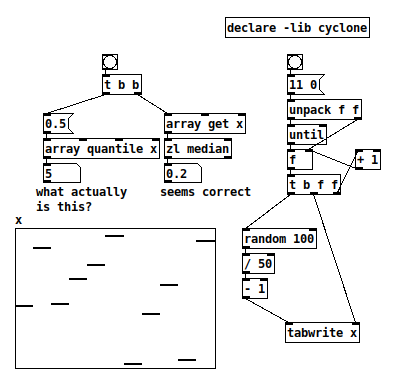• ### [array quantile]

Does anybody know what the left inlet, and only outlet, of [array quantile] represent?

The left inlet is supposed to be 0.0 - 1.0, which I assumed to be a proportion. That is, I had guessed that 0.5 --> [array quantile x] would return either a value where half the array elements are below and half above, or the index whose value meets the same criterion.

This appears not to be the case.

For example, given array values (from a concrete test):

``````-0.1 0.72 -0.08 0.28 0.48 0.9 -0.94 -0.22 0.2 -0.88 0.82
``````

0.5 --> [array quantile x] returns 5 (in other tests, it returned 2, 3, 7... so it isn't just half the array size). Assuming array indexing begins at 0, this points to 0.9.

Sorting the values gives:

``````-0.94 -0.88 -0.22 -0.1 -0.08 0.2 0.28 0.48 0.72 0.82 0.9
``````

... whereupon we find that 0.9 is the largest value in the array...?

So I'm quite puzzled. The help file says "for instance, the 0.5 quantile is the median of the array" (which should be 0.2) but this does not line up with any concrete result I have been able to obtain.

hjh20-1030-quantile-is-not-median.pd

• Posts 7 | Views 434
• @ddw_music As I understand quantile it is not median.
It finds the medians (only if you input 0.5), and returns the position (index in the sample) where the median of values before and after that position are equal ........ where the sample is divided into equal-sized, adjacent, subgroups.
David.

• @whale-av I'm afraid I'm still not following.

"Before and after that position" -- so, I split the array at the index returned by [array quantile], and there is supposed to be some property of those two arrays that will be similar or equal... it isn't clear to me what that property is.

I have a general sense of what a quantile is -- split the area under the function into regions of equal areas (so the 'x' width of each region may not be the same). But 1/ that's very different from a median and 2/ I am as yet unable to find a relationship between what Pd is doing here and this "area under the curve" definition of quantile.

For 1/ I'm concerned that the documentation is misleading.

hjh

• @ddw_music I am struggling like you for the answer.......
This looks fairly simple...... https://www.statisticshowto.com/quantile-definition-find-easy-steps/
And it should be easier to understand with a simple distribution........ quantile2.pd (not sure my first upload was correct).
Could then drag some values up and down to see the effect.
The input to [quantile] seems to be the ratio..... so 0.5 is median.
David.

• OK, so I ended up having to read the source code.

It's definitely not a statistical median.

The first difference: [array quantile] assumes all positive values. Anything negative will be clipped to 0. A traditional median requires no such assumption.

What I was looking for earlier today was a traditional median (e.g. [zl median]). So I accidentally chose to use a bipolar distribution, -1 to +1. For this range of values, [zl median] behaves well and [array quantile] will be essentially garbage.

That limitation is noted in the help file... but I was proceeding stubbornly on the assumption that the help file's earlier reference to "median" meant a statistical median. So I missed that.

So the result is the split point where the values before the split are the largest sum <= (sum of all elements * fraction). If f = 0.5, then the two array portions will have roughly equal weight.

Which is fine, but... if a user is looking for a statistical median function and does something crazy like rgrep over the documentation folder for "median" and finds "the 0.5 quantile is the median of the array," then this user is in for a surprise.

hjh

• @ddw_music Well quantile is definitely not what you want unless you can map your data into the domain it expects. But if you're curious what it could be used for, besides to make a random function with an arbitrary, table-driven probability distribution (as documented), here's a problem where I found it useful.

My mixer doubles as a control surface, however the MIDI from the faders is not very linear. It reflects the mixer's volume curve, but the function isn't analytic--the best I can do is capture it as a table. So if I want to invert that function in order to linearize the incoming MIDI, I have to find a way to invert that table. Here is the method I came up with, applied to an analytic function with a known inversion, and then to an arbitrary monotonic increasing function:
table inversion using quantile.pd
table inversion using quantile 2.pd

• I'm not saying that quantile isn't useful -- only that the help shouldn't call 0.5-ile a median when it isn't.

But that won't be solved here, so I'll open a bug for it.

hjh

Posts 7 | Views 434
Internal error.

Oops! Looks like something went wrong!